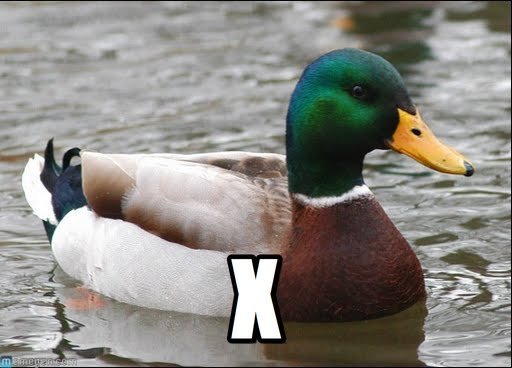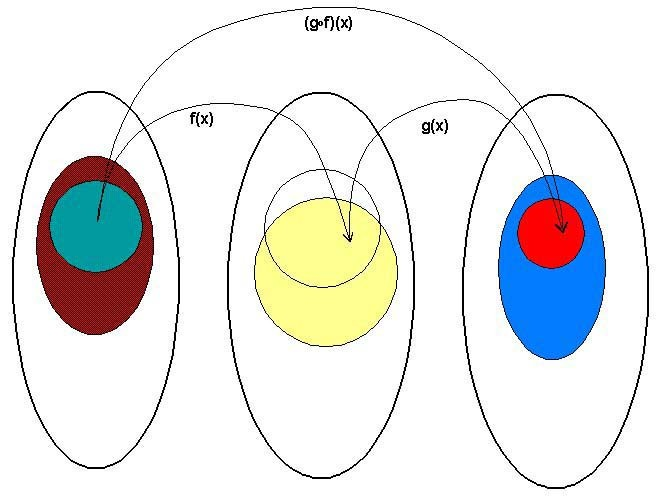## Preface## Lambdalambda包括一条变换规则（变量替换）和一条函数定义方式，Lambda演算之通用在于，任何一个可计算函数都能用这种形式来表达和求值。因而，它是等价于图灵机的.1 multiple(x, y) = x*y


1 (x,y) -> x*y


1. lambda 接受第一个参数 5, 返回另一个 lambda
1   (5) -> (y -> 5*y)

2. 该返回的 lambda y -> 5*y 接收 y 并且返回 5*y, 若在用 4 调用该 lambda
1   4 -> 5*4


### 箭头函数(arrow function)

#### 声明一个箭头函数

1 ([param] [, param]) => {
2    statements
3 }
4 // or
5 param => expression


#### 为什么要用箭头函数

1 var a = [1,2,3,4,5];
2 a.map(function(x){ return x*2 });


1 a.map(x => x*2);


map(x => x*2) 要更 make sense, 因为我们需要的匿名函数只需要做一件事情, 我们需要的是 一个函数 f, 可以将给定 x, 映射到 y. 翻译这句话的最简单的方式不就是 f  (x > x*2)

#### Lexical this

 1       var Multipler = function(inc){
 2         this.inc = inc;
 3       }
 4       Multipler.prototype.multiple = function(numbers){
 5         return numbers.map(function(number){
 6           return this.inc * number;
 7         })
 8       }
 9       new Multipler(2).multiple([1,2,3,4])
10   // => [NaN, NaN, NaN, NaN]  不 work, 因为 map 里面的 this 指向的是全局变量( window)
11
12       Multipler.prototype.multiple = function(numbers){
13         var self = this; // 保持 Multipler 的 this 的缓存
14         return numbers.map(function(number){
15           return self.inc * number;
16         })
17       }
18       new Multipler(2).multiple([1,2,3,4]) // => [ 2, 4, 6, 8 ]


1   Multipler.prototype.multiple = function(numbers){
2     return numbers.map((number) => number*this.inc);
3   };
4
5   console.log(new Multipler(2).multiple([1,2,3,4]));// => [ 2, 4, 6, 8 ]


### JavaScript的匿名函数(anonymous function)

#### 创建一个匿名函数

1   function(x){
2       return x*x;
3   }// => SyntaxError: function statement requires a name


1   var a = new Array() // new Array 是表达式, 而这整行叫语句 statement


1   var squareA = function(x){
2       return x*x;
3   }


1 console.log(squareA) // => function squareA()


1   function squareB(x){
2       return x*x;
3   } // => undefined


squareB 用的是 function statement 直接声明(显然 statement 没有返回), 而 squareA 则是先用 function expression 创建一个匿名函数, 然后将返回的函数赋给了名为 squareA 的变量. 因为表达式是有返回的:

1   console.log(function(x){ return x*x});
2   // => undefined
3   // => function ()


#### 使用匿名函数

JavaScript 的函数是一等函数. 这意味着我们的函数跟值的待遇是一样的,于是它

1   var square = function(x) {return x*x}


1   console.log(function(x){return x*x})


1   function multiply(x){
2       return function(y){
3           return x*y;
4       }
5   }
6   multiply(1)(2) // => 2

1. 第二章会详细解释高阶函数和闭包.
2. 柯里化会在第二章详细讨论.
3. 可以看看es6比较有意思的新特性 http://blog.oyanglul.us/javascript/essential-ecmascript6.html
4. Chrome有一个 feature toggle 可以打开部分 es6 功能 <chrome://flags/#enable-javascript-harmony>

## 高阶函数(Higher-order function)

1 f(x) => (y -> x(y))
2 (y) => x(y)### Higher-order function

#### 函数作为参数

1   [1,3,2,5,4].sort( (x, y) => x - y )


1   [{id:1, name:'one'},
2    {id:3, name:'three'},
3    {id:2, name:'two'},
4    {id:5, name:'five'},
5    {id:4, name:'four'}].sort((x,y) => x.id - y.id)


#### 函数作为返回值

Note 听起来很怪不是吗, 函数怎么有方法, 实际上 JavaScript 的 function 是一个特殊 对象, 试试在 Firefox console 里敲 console.log. 是不是看到了一些方法, 但是 typeof console.log 是 function

1   var E = () => {}
2   var aliasFor = oldName => {
3       var fn = newName => {
4         E[newName] = E[oldName];
5         return fn;
6       };
7       return (fn.is = fn.are = fn.and = fn);
8   };1   aliasFor('reduce').is('reduceLeft').is('foldl')


### 柯里化 currying1   max 3 4
2   (max 3) 4


#### 我们来看一个问题

##### 1. 写一个函数, 可以连接字符数组, 如 f(['1','2']) => '12'

1 var concatArray = function(chars){
2   return chars.reduce(function(a, b){
3     return a.concat(b);
4   });
5 }
6 concat(['1','2','3']) // => '123'


##### 2. 现在我要其中所有数字加1, 然后在连接
1 var concatArray = function(chars, inc){
2   return chars.map(function(char){
3     return (+char)+inc + '';
4   }).reduce(function(a,b){
5       return a.concat(b)
6   });
7 }
8 console.log(concatArray(['1','2','3'], 1))// => '234'

##### 3. 所有数字乘以2, 再重构试试看
 1 var multiple = function(a, b){
 2   return +a*b + ''
 3 }
 4 var concatArray = function(chars, inc){
 5   return chars.map(function(char){
 6     return multiple(char, inc);
 7   }).reduce(function(a,b){
 8       return a.concat(b)
 9   });
10 }
11 console.log(concatArray(['1','2','3'], 2)) // => '246'


#### 柯里化函数接口

 1 var multiple = function(a){
 2   return function(b){
 3     return +b*a + ''
 4   }
 5 }
 6
 7 var plus = function(a){
 8   return function(b){
 9     return (+b)+a + ''
10   }
11 }
12 var concatArray = function(chars, stylishChar){
13   return chars.map(stylishChar)
14     .reduce(function(a,b){
15       return a.concat(b)
16   });
17 }
18 console.log(concatArray(['1','2','3'], multiple(2)))
19 console.log(concatArray(['1','2','3'], plus(2)))


1 concatArray(['1','2','3'], multiple(2))


1 chars.map(stylishChar)


#### 自动柯里化

1   max 3 4


1 (max 3) 4


1   ghci> :t max
2   max :: Ord a => a -> a -> a


1 ghci> :t max 3
2 (Num a, Ord a) => a -> a


1   function willNotCurry(a, b, c) {
2       console.log(a, b, c)
3       return a*b-c;
4   }
5   willNotCurry(1)
6   // => NaN
7   // => 1 undefined undefined


1 var multiple = curry(function(a, b){
2   return +b*a + ''
3 })
4 var plus = curry(function(a, b){
5   return (+b)+a + ''
6 })


### 函数组合 function composition#### pipe

1 find / | grep porno


1   _(data)
2     .chain()
3     .map(data1,fn1)
4     .filter(data2, fn2)
5     .value()


1   pipe(
2     map(fn1),
3     filter(fn2)
4   )(data)